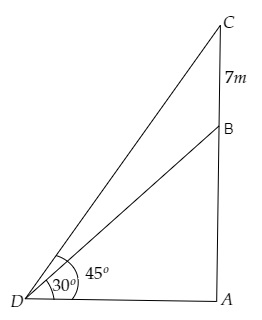# A vertical tower stands on a horizontal plane and is surmounted by a flag-staff of height $7 \mathrm{~m}$. From a point on the plane, the angle of elevation of the bottom of the flag-staff is $30^{\circ}$ and that of the top of the flag-staff is $45^{\circ}$. Find the height of the tower.

Given:

A vertical tower stands on a horizontal plane and is surmounted by a flag-staff of height $7 \mathrm{~m}$.

From a point on the plane, the angle of elevation of the bottom of the flag-staff is $30^{\circ}$ and that of the top of the flag-staff is $45^{\circ}$.

To do:

We have to find the height of the tower.

Solution:Let $AB$ be the tower and $BC$ be the length of the flag-staff.

Let point $D$ be the point of observation.

From the figure,

$\mathrm{BC}=7 \mathrm{~m}, \angle \mathrm{BDA}=30^{\circ}, \angle \mathrm{CDA}=45^{\circ}$

Let the height of the tower be $\mathrm{DB}=h \mathrm{~m}$ and the distance between the tower and point $D$ be $\mathrm{AD}=x \mathrm{~m}$.

This implies,

$\mathrm{AC}=x+h \mathrm{~m}$

We know that,

$\tan \theta=\frac{\text { Opposite }}{\text { Adjacent }}$

$=\frac{\text { CA }}{DA}$

$\Rightarrow \tan 45^{\circ}=\frac{h+7}{x}$

$\Rightarrow 1=\frac{h+7}{x}$

$\Rightarrow h+7=x(1) \mathrm{~m}$

$\Rightarrow x=h+7 \mathrm{~m}$...........(i)

Similarly,

$\tan \theta=\frac{\text { Opposite }}{\text { Adjacent }}$

$=\frac{\text { BA }}{DA}$

$\Rightarrow \tan 30^{\circ}=\frac{h}{x}$

$\Rightarrow \frac{1}{\sqrt3}=\frac{h}{h+7}$                 [From (i)]

$\Rightarrow h+7=h\sqrt3 \mathrm{~m}$

$\Rightarrow h(\sqrt3-1)=7 \mathrm{~m}$

$\Rightarrow h(1.732-1)=7 \mathrm{~m}$

$\Rightarrow h=\frac{7}{0.732} \mathrm{~m}$

$\Rightarrow h=9.56 \mathrm{~m}$

Therefore, the height of the tower is $9.56 \mathrm{~m}$.

Updated on: 10-Oct-2022

40 Views